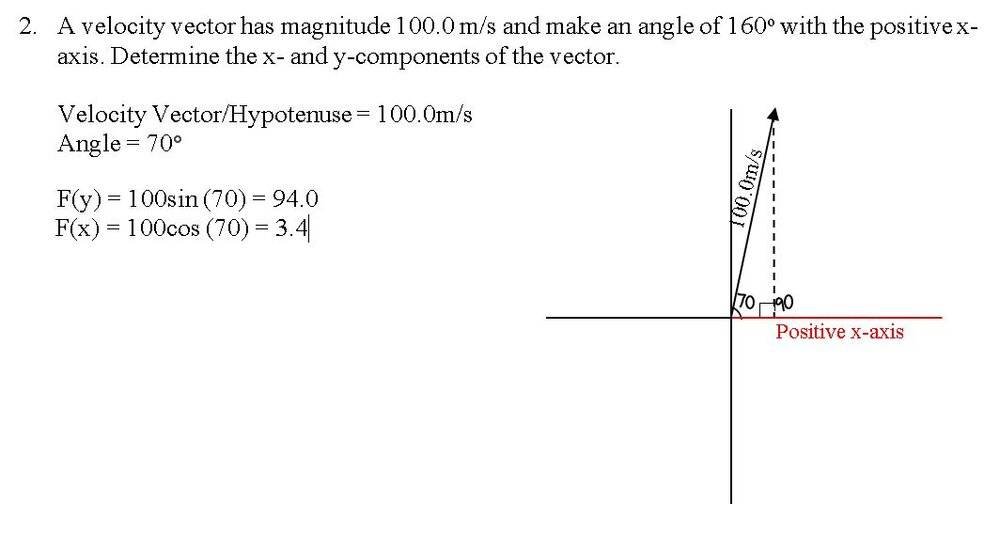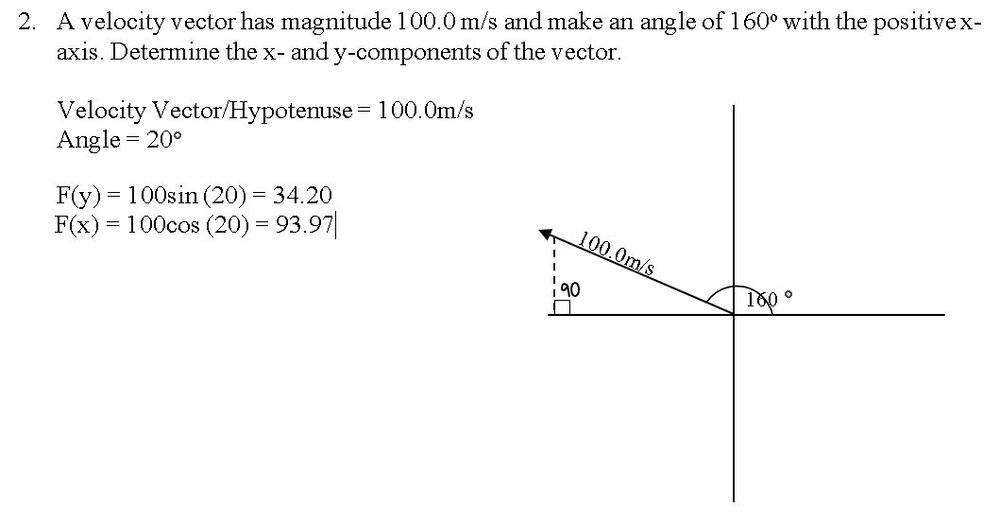# Finding the components of this velocity vector

In summary, the problem is asking to determine the x- and y-components of a velocity vector with a magnitude of 100.0 m/s and an angle of 160° with the positive x-axis. The correct sketch for this would be on the second quadrant. The confusion may have arisen from the use of "positive x-axis" in the problem. The angle should be measured counterclockwise from the horizontal x-axis, making the x-coordinate negative. The correct equations to use are ##\sin(\pi-x)=\sin x## and ##\cos(\pi-x)=-\cos x##.

Homework Statement
A velocity vector has magnitude 100.0 m/s and make an angle of 160° with the positive x-axis. Determine the x- and y-components of the vector.
Relevant Equations
Trig functionsHomework Statement:: A velocity vector has magnitude 100.0 m/s and make an angle of 160° with the positive x-axis. Determine the x- and y-components of the vector.
Relevant Equations:: Trig functions

View attachment 269386
Welcome to PhysicsForums.That sketch looks wrong. What line is 180 degrees from the x-axis? So 160 degrees would be pretty close to that line, not vertical like that...

Just started Physics and I wasn't sure if my answer and solution was right. I attempted to project it on the cartesian plane based on my understanding. It said "an angle of 160 with the positive x-axis." What I understood from this is that angles of the triangle that makes contact to the x-axis equal to 160 so I assumed the angles would be 70 and 90.

berkeman said:
Welcome to PhysicsForums.That sketch looks wrong. What line is 180 degrees from the x-axis? So 160 degrees would be pretty close to that line, not vertical like that...
Hi! What would the correct sketch look like? While I was sketching it I knew something was fishy and I just had to ask hahaha

Just started Physics and I wasn't sure if my answer and solution was right. I attempted to project it on the cartesian plane based on my understanding. It said "an angle of 160 with the positive x-axis." What I understood from this is that angles of the triangle that makes contact to the x-axis equal to 160 so I assumed the angles would be 70 and 90.
No, usually the angle is measured counterclockwise from the horizontal x-axis. So an angle of 90 degrees is straight up (along the y-axis), and an angle of 180 degrees is pointing left along the -x-axis. So where would 160 degrees counterclockwise from the x-axis be? And what would the x- and y- components of that vector be then?

•Physics4Funn
•Lnewqban
berkeman said:
So it would then be on the 2nd Quadrant, correct? What confused me was the usage of "positive x-axis" so I wrongfully assumed that it would be strictly in the 1st Quadrant (where x values are positive). I will solve it and send my answer! :)